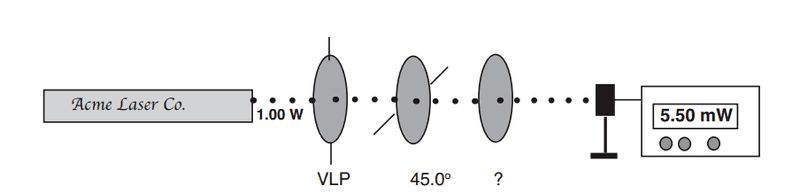# Polarizers in series, I got the correct answer, want to verify why

## Homework StatementAn unpolarized laser emits 1.00 W of continuous optical power.
It's beam is directed into three identical linear polarizers, each with a maximum transmission of 95.0%. The first polarizer has its transmission axis vertical, the second at 45.0 from the vertical, and the third is in an unknown orientation. An optical power meter reads the overall power transmitted as 5.50 milliwatts. Find the orientation of the third polarizer's transmission axis relative to the vertical. (20 points)

## Homework Equations

I = Imax*1/2 * cos212)

## The Attempt at a Solution

So I set up the equation as such:
5.5*10-3= (0.95)3*1.0W*1/2 * cos2(90°-45°)*cos2(θ - 45°)

I do some algebra and get θ = 125.78, which is the correct answer.

What I'm looking to clarify is a few things.

For the (0.95)3 part of the equation, the exponent will always just be the number of polarisers if they all have the same maximum transmission?

cos2(θ - 45°), the -45° comes from the previous polariser right? So if there was another polariser that had an angle 60° from the vertical place before the last unknown polariser, the equation would change to:
5.5*10-3= (0.95)4*1.0W*1/2 * cos2(45°)*cos2(60°-45°)*cos2(θ - (60°-45°))

Is this correct? I'm not 100% certain and I don't have access to any more problems in the same form as this one that I have the answer to.

Thank you in advance.

## Answers and Replies

mfb
Mentor
For the (0.95)3 part of the equation, the exponent will always just be the number of polarisers if they all have the same maximum transmission?

cos2(θ - 45°), the -45° comes from the previous polariser right?
Right
So if there was another polariser that had an angle 60° from the vertical place before the last unknown polariser, the equation would change to:
5.5*10-3= (0.95)4*1.0W*1/2 * cos2(45°)*cos2(60°-45°)*cos2(θ - (60°-45°))
I think the angles should be 45°, 15° (=60-45) and θ-60°, as θ is measured relative to the vertical axis.